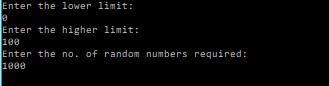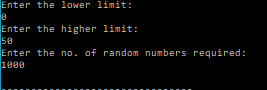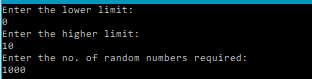# Central Limit Theorem Demonstration – C PROGRAM

One of the fundamental theorems of probability is the Central Limit Theorem. This theorem says that if$S_n$ is the sum of$n$ mutually independent random variables, then the distribution function of$S_n$, for a large$n$, is well-approximated by a certain type of continuous function known as a normal density function, which is given by the formula$f_{\mu, \sigma}(x)=\frac{1}{\sqrt{2 \pi} \sigma} e^{-(x-\mu)^{2} /\left(2 \sigma^{2}\right)}$

We will demonstrate this using a C program and the following problems.

#### Distribution of Sum of Random Variables:

Case 1
1. Let M_i = a_i be marks of n students in one subject, i=1,2,…n (n=1000).
2. Let a_i be the uniformly distributed random numbers between 0 and m (m is the maximum
marks=100).
3. Find the frequency distribution of M.

CODE:

/**********************************************
****Random Marks Frequency Distribution***
***********************************************/
#include<stdio.h>
#include<math.h>
/**Function that generates a random number.
Parameters:
r0: initial (first) seed
a: scale factor , so that a*r0 give the first random number
m: gives the max. value of random numbers that can be generated (m-1)
c: additional displacement(offset) factor
**/
int rand(int r0, int a, int m, int c){
int r1=(a*r0+c)%m;
return r1;
}
/**Function that generates random numbers in a given range: [min,max], given a seed r0, and stores them in an array that is passed as an argument.
Parameters:
r0: initial (first) seed
a: scale factor , so that a*r0 give the first random number
m: gives the max. value of random numbers that can be generated (m-1)
c: additional displacement factor
n: no. of random numbers to be generated
x[n]: array that will store the random numbers
min: lower limit for random nos.
max: upper limit for random nos.
**/
void randomNos(int r0, int a, int m, int c, int n, int x[n], int min, int max){
int r1=rand(r0,a,m,c);
int r2=min+((max-min+1)*r1)/m;
int i;
for(i=0;i<n;i++){
x[i]=r2;
r1=rand(r1,a,m,c);
r2=min+((max-min+1)*r1)/m;
}
}
main(){
int min, max, n, a=1093, m=86436, c=18257, r0=43;
printf("Enter the lower limit:\n");
scanf("%d",&min);
printf("Enter the higher limit:\n");
scanf("%d",&max);
printf("Enter the no. of random numbers required:\n");
scanf("%d",&n);
int random[n];
randomNos(r0, a, m, c, n, random, min, max);
FILE *fp=NULL;
fp=fopen("marks.txt","w");
int i,j;
//printf("The random numbers between %d and %d are:\n",min, max);
for(i=0;i<n;i++){
fprintf(fp,"%d\n",random[i]);
}
//Begin distribution calculations within different intervals
int h=10; //width of interval
int count; //10 intervals of width 10
for(j=0;j<10;j++){
count[j]=0;
for(i=0;i<n;i++){
if(j!=9){
//find out the number of randomnumbers within an interval
if((j*h<=random[i])&&(random[i]<(j+1)*h)){
count[j]++;  //find out the number of randomnumbers within an interval
}
} else{
//find out the number of randomnumbers within an interval
if((j*h<=random[i])&&(random[i]<=(j+1)*h)){
count[j]++;  //find out the number of randomnumbers within an interval
}
}

}
}
FILE *fp2=NULL;
fp2=fopen("randMarksDistribution.txt","w");
for(i=0;i<10;i++){
fprintf(fp2,"%d\t%d\n",i*h,count[i]);
//printf("%d\n",count[i]);
}

}Case 2
1. Let M_i = a_i + +b_i be total marks of n students in TWO subjects, i=1,2,…n (n=1000).
2. Let each of a_i and b_i be the uniformly distributed random numbers between 0 and m (m is the
maximum marks of each subject=50).
3. Find the frequency distribution of M.

CODE:

/**********************************************
****Random Marks Frequency Distribution***
***********************************************/
#include<stdio.h>
#include<math.h>
/**Function that generates a random number.
Parameters:
r0: initial (first) seed
a: scale factor , so that a*r0 give the first random number
m: gives the max. value of random numbers that can be generated (m-1)
c: additional displacement(offset) factor
**/
int rand(int r0, int a, int m, int c){
int r1=(a*r0+c)%m;
return r1;
}
/**Function that generates random numbers in a given range: [min,max], given a seed r0, and stores them in an array that is passed as an argument.
Parameters:
r0: initial (first) seed
a: scale factor , so that a*r0 give the first random number
m: gives the max. value of random numbers that can be generated (m-1)
c: additional displacement factor
n: no. of random numbers to be generated
x[n]: array that will store the random numbers
min: lower limit for random nos.
max: upper limit for random nos.
**/
void randomNos(int r0, int a, int m, int c, int n, int x[n], int min, int max){
int r1=rand(r0,a,m,c);
int r2=min+((max-min+1)*r1)/m;
int i;
for(i=0;i<n;i++){
x[i]=r2;
r1=rand(r1,a,m,c);
r2=min+((max-min+1)*r1)/m;
}
}
main(){
int min, max, n, a=1093, m=86436, c=18257, r0=43;
printf("Enter the lower limit:\n");
scanf("%d",&min);
printf("Enter the higher limit:\n");
scanf("%d",&max);
printf("Enter the no. of random numbers required:\n");
scanf("%d",&n);
int ai[n];
int bi[n];
int Marks[n];
randomNos(0, a, m, c, n, ai, min, max);
randomNos(2000, a, m, c, n, bi, min, max);
FILE *fp=NULL;
fp=fopen("marks2.txt","w");
int i,j;
//printf("The random numbers between %d and %d are:\n",min, max);
for(i=0;i<n;i++){
Marks[i]=ai[i]+bi[i];
fprintf(fp,"%d\n",Marks[i]);
}
//Begin distribution calculations within different intervals
int h=5; //width of interval
int count; //10 intervals of width 10
for(j=0;j<20;j++){
count[j]=0;
for(i=0;i<n;i++){
if(j!=19){
//find out the number of randomnumbers within an interval
if((j*h<=Marks[i])&&(Marks[i]<(j+1)*h)){
count[j]++;  //find out the number of randomnumbers within an interval
}
} else {
//find out the number of randomnumbers within an interval
if((j*h<=Marks[i])&&(Marks[i]<=(j+1)*h)){
count[j]++;  //find out the number of randomnumbers within an interval
}
}
}
}
FILE *fp2=NULL;
fp2=fopen("randMarksDistribution2.txt","w");
for(i=0;i<20;i++){
fprintf(fp2,"%d\t%d\n",i*h,count[i]);
//printf("%d\n",count[i]);
}

}Case 3
1. Let M_i = a_i + +b_i +c_i + d_i + e_i + f_i + g_i +h_i +j_i + k_i be total marks of n students in TEN
subjects, i=1,2,…n (n=1000).
2. Let each of a_i, b_i, c_i, ……k_i be the uniformly distributed random numbers between 0 and m (m is
the maximum marks of each subject=10).
3. Find the frequency distribution of M.

CODE:

/**********************************************
****Random Marks Frequency Distribution***
***********************************************/
#include<stdio.h>
#include<math.h>
/**Function that generates a random number.
Parameters:
r0: initial (first) seed
a: scale factor , so that a*r0 give the first random number
m: gives the max. value of random numbers that can be generated (m-1)
c: additional displacement(offset) factor
**/
int rand(int r0, int a, int m, int c){
int r1=(a*r0+c)%m;
return r1;
}
/**Function that generates random numbers in a given range: [min,max], given a seed r0, and stores them in an array that is passed as an argument.
Parameters:
r0: initial (first) seed
a: scale factor , so that a*r0 give the first random number
m: gives the max. value of random numbers that can be generated (m-1)
c: additional displacement factor
n: no. of random numbers to be generated
x[n]: array that will store the random numbers
min: lower limit for random nos.
max: upper limit for random nos.
**/
void randomNos(int r0, int a, int m, int c, int n, int x[n], int min, int max){
int r1=rand(r0,a,m,c);
int r2=min+((max-min+1)*r1)/m;
int i;
for(i=0;i<n;i++){
x[i]=r2;
r1=rand(r1,a,m,c);
r2=min+((max-min+1)*r1)/m;
}
}
main(){
int min, max, n, a=1093, m=86436, c=18257, r0=43;
printf("Enter the lower limit:\n");
scanf("%d",&min);
printf("Enter the higher limit:\n");
scanf("%d",&max);
printf("Enter the no. of random numbers required:\n");
scanf("%d",&n);
int A[10*n];
int Marks[n];
randomNos(r0, a, m, c, 10*n, A, min, max);
FILE *fp=NULL;
fp=fopen("marks3.txt","w");
int i,j;
//printf("The random numbers between %d and %d are:\n",min, max);
for(j=0;j<n;j++){
Marks[j]=0;
for(i=j;i<10*n;i=i+n){
Marks[j]=Marks[j]+A[i];
}
fprintf(fp,"%d\n",Marks[j]);
}
//Begin distribution calculations within different intervals
int h=5; //width of interval
int count; //10 intervals of width 100
for(j=0;j<20;j++){
count[j]=0;
for(i=0;i<n;i++){
if(j!=19){
//find out the number of randomnumbers within an interval
if((j*h<=Marks[i])&&(Marks[i]<(j+1)*h)){
count[j]++;  //find out the number of randomnumbers within an interval
}
} else {
//find out the number of randomnumbers within an interval
if((j*h<=Marks[i])&&(Marks[i]<=(j+1)*h)){
count[j]++;  //find out the number of randomnumbers within an interval
}
}

}
}
FILE *fp2=NULL;
fp2=fopen("randMarksDistribution3.txt","w");
for(i=0;i<20;i++){
fprintf(fp2,"%d\t%d\n",i*h,count[i]);
//printf("%d\n",count[i]);
}

}Through the above problems, it’s pretty much apparent that as the number of random variables whose sum is being taken increases their distribution tends towards the normal (Gaussian) distribution.

### References:

https://en.wikipedia.org/wiki/Central_limit_theorem
https://www.investopedia.com/terms/c/central_limit_theorem.asp
https://www.dartmouth.edu/~chance/teaching_aids/books_articles/probability_book/Chapter9.pdf[wpedon id="7041" align="center"]